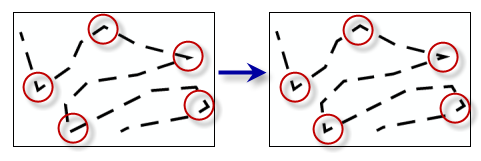# Set Control Point By Angle (Cartography)

## Summary

Places a control point at vertices along a line or polygon outline where the angle created by a change in line direction is less than or equal to a specified maximum angle.

## IllustrationDash placement can be improved along a line or polygon outline when control points are placed at prominent angles.

## Usage

##### Caution:

This tool modifies the input data. See Tools that do not create output datasets for more information and strategies to avoid undesired data changes.

• Control points cannot be added to shapefiles.

## Syntax

`SetControlPointByAngle(in_features, maximum_angle)`
 Parameter Explanation Data Type in_features The feature layer containing line or polygon features. Feature Layer maximum_angle The angle used to determine whether or not a vertex along a line or polygon outline will be set as a control point. The angle value must be greater than zero and less than 180 decimal degrees. Double

#### Derived Output

 Name Explanation Data Type out_representations The updated input features. Feature Layer

## Code sample

SetControlPointByAngle example 1 (Python window)

The following Python window script demonstrates how to use the SetControlPointByAngle tool in immediate mode.

``````import arcpy
arcpy.env.workspace = "C:\data"

arcpy.SetControlPointByAngle_cartography("trails.lyrx", "135")``````
SetControlPointByAngle example 2 (stand-alone Python script)

This stand-alone script shows an example of using the SetControlPointByAngle tool.

``````# Name: SetControlPointByAngle_standalone_script.py
# Description: Places a control point at vertices along a line or polygon
#              outline where the angle created by a change in line direction is
#              less than or equal to a specified maximum angle

# Import system modules
import arcpy

# Set environment settings
arcpy.env.workspace = "C:/data"

# Set local variables
in_layer = "trails.lyrx"
minimum_angle_deviation = "135"

# Execute Set Representation Control Point At Intersect
arcpy.SetControlPointByAngle_cartography(in_layer, minimum_angle_deviation)``````

• Basic: No
• Standard: No